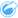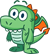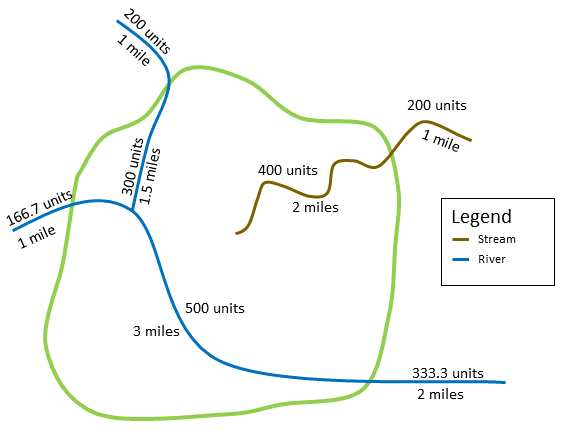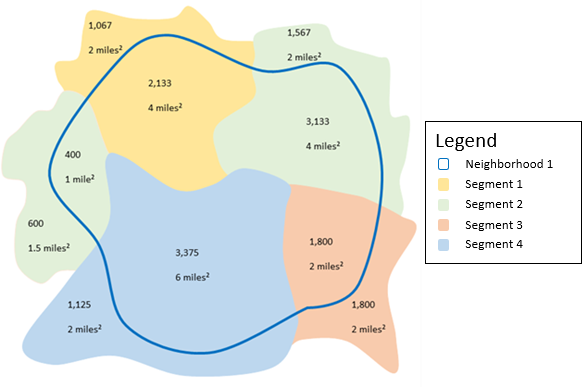# Spatial Aggregation

Spatial Aggregation calculates statistics in areas where an input layer overlaps a boundary layer.

## Example

A business analyst for a consortium of colleges is doing research for a marketing campaign in states with high-value colleges and wants to know which state has the most colleges with a high return on investment (ROI). Spatial Aggregation can be used to aggregate the colleges into states to find the number of colleges with above average ROI.

## Use Spatial Aggregation

Spatial Aggregation can be run on maps with two layers: one area layer with the boundaries that will be used for aggregation (for example, counties, census tracts, or police districts) and one layer to aggregate.

Complete the following steps to run Spatial Aggregation:

1. If necessary, click the map card to activate it. A card is active when the toolbar and Action buttonappear.
2. Click the Action button, then choose Spatial Aggregation.
3. For Choose area layer, select the boundary layer. For Choose layer to summarize, select the layer to aggregate.
4. For Style by, select the field or statistic that you want to calculate and display. Use Additional options to select additional fields and statistics, if necessary.
5. Click Run.
##### Tip:Spatial Aggregation can also be run by dragging a dataset onto the Spatial aggregation drop zone on an existing map.

## Usage notes

The Choose area layer and Choose layer to summarize parameters are used to select the boundary layer and the layer that will be summarized. For the Choose area layer parameter, only layers with area features will be available.

The Style by parameter can be used to change the statistic being calculated. The default statistic depends on the type of layer being summarized. The drop-down menu can be used to select a different style option. The following table summarizes the Style by options for each layer type:

Summary layer typeDefault style optionOther style options

Point

Count

Number or rate/ratio field (sum, minimum, maximum, average, or mode)

String fields (mode)

Line

Number (sum) or rate/ratio (average) field

Number or rate/ratio field (sum, minimum, maximum, average, or mode)

String fields (mode)

Sum of length (meters, kilometers, feet, or miles)

Area

Number (sum) or rate/ratio (average) field

Number or rate/ratio field (sum, minimum, maximum, average, or mode)

String fields (mode)

Sum of area (square meters, square kilometers, square feet, or square miles)

##### Note:

It is best practice to use numbers rather than rate/ratios when calculating statistics for lines and areas so that the proportional calculations make logical sense. For more information, see How Spatial Aggregation works.

The Additional options parameter can be expanded and extra statistics can be assigned. Each time a field is added to the list of summary statistics, a new field will appear below it.

## Limitations

When you perform spatial aggregation or spatial filtering on data from the same database connection, you must ensure that all the data is stored in the same spatial reference system. For datasets from SQL Server, the data must also have the same data type (geography or geometry).

The following limitations apply for Google BigQuery, Snowflake, and database platforms that are not supported out-of-the-box:

• Spatial Aggregation using line and area features as the Choose a layer to summarize parameter is not supported for read-only connections.
• Both input layers must be from the same database connection.

Google BigQuery does not support mode calculations.

## How Spatial Aggregation works

Average statistics are calculated using weighted mean for line and area features. The following equation is used to calculate weighted mean:

``````where:
N = number of observations
xi = observations
Wi = weights``````

### Points

Point layers are summarized using only the point features within the input boundary. Therefore, none of the calculations are weighted.

The figure and table below explain the statistical calculations of a point layer within a hypothetical boundary. The Population field was used to calculate the numeric statistics (count, sum, minimum, maximum, and average) and the Type field was used for mode.

FieldStatisticResult District AResult District B

Population

Count

6

6

Sum

``````280 + 408 + 356 + 361 + 450 + 713
= 2,568``````
``````370 + 422 + 495 + 607 + 574 + 932
= 3,400``````

Minimum

Minimum of:

``````[280 408 356 361 450 713]
= 280``````

Minimum of:

``````[370 422 495 607 574 932]
= 370``````

Maximum

Maximum of:

``````[280 408 356 361 450 713]
= 713``````

Maximum of:

``````[370 422 495 607 574 932]
= 932``````

Average

``````2,568/6
= 428``````

``````3,400/6
= 566.67``````

Type

Mode

Primary School

Primary School

A real-life scenario in which this analysis could be used is in determining the total number of students in each school district. Each point represents a school. The Type field gives the type of school (elementary, middle school, or secondary) and a student population field gives the number of students enrolled at each school. The calculations and results are given in the table above. From the results you can see that District A has 2,568 students and District B has 3,400 students.

### Lines

Line layers are summarized numerically using only the proportions of the line features that are within the input boundary. When summarizing lines, use fields with counts and amounts rather than rates or ratios so proportional calculations make logical sense in your analysis. The results are displayed using graduated symbols.

The mode for line layers is based on the count of features that intersect the boundary. Lines do not have to be completely contained within a boundary to be counted toward the mode and each line is counted as one feature, regardless of the proportion that is contained within the boundary. The results are displayed using unique symbols.

The figure and table below explain the statistical calculations of a line layer within a hypothetical boundary. The volume was used to calculate the statistics (sum, minimum, maximum, and average) for the layer. The statistics are calculated using only the proportion of the lines that are within the boundary. The mode is calculated for the type of water feature.StatisticFieldResult

Sum of length

Length

``2 miles + 3 miles + 1.5 miles = 6.5 miles``
##### Note:

Length can also be calculated in feet, meters, and kilometers.

Sum

Volume

``400 + 500 +300 = 1,200``

Minimum

Minimum of:

``[400 500 300] = 300``

Maximum

Maximum of:

``[400 500 300] = 500``

Average

``````((2*400)+(3*500) + (1.5*300))/(2+3+1.5)
= 423.08``````

Mode

Type

River

A real-life scenario in which this analysis could be used is in determining the total volume of water in rivers within the boundaries of a state park. Each line represents a river that is partially located inside the park. From the results, you can see that there are 6.5 miles of rivers within the park and the total volume is 1,200 units.

### Areas

Area layers are summarized using only the proportions of the area features that are within the input boundary. When summarizing areas, use fields with counts and amounts rather than rates or ratios so proportional calculations make logical sense in your analysis. The results are displayed using graduated colors.

The mode for area layers is based on the count of features that intersect the boundary. Areas do not have to be completely contained within a boundary to be counted toward the mode and each area is counted as one feature, regardless of the proportion that is contained within the boundary. The results are displayed using unique symbols.

The figure and table below explain the statistical calculations of an area layer within a hypothetical boundary. The populations were used to calculate the statistics (sum, minimum, maximum, and average) for the layer. The statistics are calculated using only the proportion of the area that is within the boundary. The mode is calculated using the tapestry segment designation for each area.StatisticFieldResult

Sum of area

Area

``4 mi2 + 4 mi2 + 1 mi2 + 6 mi2 + 2 mi2 = 17 mi2``
##### Note:

Area can also be calculated in square feet, square meters, and square kilometers.

Sum

Population

``2,133 + 3,133 + 400 + 3,375 + 1,800 = 10,841``

Minimum

Minimum of:

``[2,133 3,133 400 3,375 1,800] = 400``

Maximum

Maximum of:

``[2,133 3,133 400 3,375 1,800] = 3,375``

Average

``````((4*2,133)+(4*3,133)+(2*1,800)+(6*3,375)+(1*400))/(4+4+2+6+1)
= 2,665.53``````

Mode

Segment

Segment 2

A real-life scenario in which this analysis could be used is in determining the population in a city neighborhood. The blue outline represents the boundary of the neighborhood and the smaller areas represent census blocks. From the results, you can see that there are 10,841 people in the neighborhood and an average of approximately 2,666 people per census block.